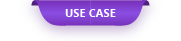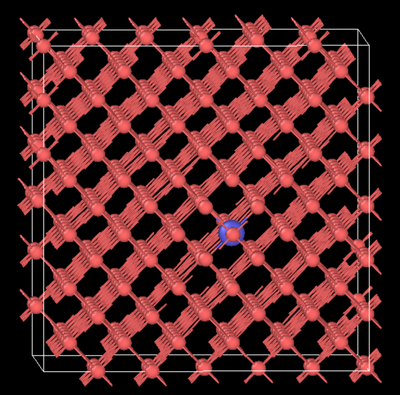Nanosecond-order analysis is the limit of ordinary molecular dynamics methods, but various accelerated molecular dynamics methods ...

# Long-term simulation using accelerated molecular dynamics

Nanosecond-order analysis is the limit of ordinary molecular dynamics methods, but various accelerated molecular dynamics methods that accelerate the time scale have been proposed as countermeasures for problems with longer time scales . .. This method can be expected to be applied to various problems such as defect / impurity diffusion and transition generation in semiconductors / dielectrics, batteries, and steel materials, element diffusion at surfaces / interfaces, and crystal growth. Here, we verified carbon diffusion in α-iron as an example that requires long-term simulation. Carbon diffusion in α-iron takes about 0.1 seconds at room temperature to move between adjacent sites at the center of the octahedron, so diffusion cannot be reproduced by the normal MD method, but long-term simulation can be performed by using the accelerated molecular dynamics method. It became possible, and we were able to confirm the state of carbon diffusion in α-iron.

#### 1. Calculation model and calculation conditions

Table 1 Calculation conditions

The model and calculation conditions used for the calculation are shown on the left. This time, we used temperature accelerated dynamics (TAD) as the accelerated molecular dynamics method . In the TAD method, he accelerates the simulation by sampling the atomic arrangement from a short-time high-temperature simulation and calculating the transition probability between each atomic arrangement. In this simulation, the temperature, which is about twice the melting point of iron, was set as a high temperature condition. All calculations were performed using the molecular dynamics calculation code LAMMPS.

#### 2. Calculation result

On the left is an MD snapshot at 423K (150 ° C). It has been confirmed that carbon atoms jump between the centers of the octahedrons of α-iron.

Table 2 Temperature and average jump time

Shows the average jump time at each simulation temperature. Using the results of five simulations with different initial conditions, the average value of the time it takes for a carbon atom to jump to the center of the octahedron of α-iron was evaluated and used as the average jump time. Since the average jump time is about several microseconds at low temperatures, it can be seen that time-scale simulations that cannot be reproduced by the normal MD method can be performed.

In addition, the calculation results of the mean square displacement and diffusion coefficient of carbon atoms are shown. It can be seen that the calculation result of the diffusion coefficient can reproduce the measured value . Since it is difficult to simulate such elemental diffusion at low temperatures with ordinary MD calculations, this method is extremely useful.

#### 3. Fields of application of this method

This time, we introduced examples of application of accelerated molecular dynamics in the steel field, but we can expect applications in other phenomena and fields that require long-term simulation such as transition generation and defect migration. The following is an example of the fields to which this method can be applied.

(1) Diffusion of impurities and defects in semiconductors and dielectric materials

(2) Ion diffusion in battery material (Ion diffusion at actual operating temperature, which is difficult to evaluate by conventional MD calculation, can be evaluated)

③ Element diffusion and crystal growth on the surface / interface

### 4. References

 Arthur F Voter, Francesco Montalenti, Timothy C Germann. “Extending the time scale in atomistic simulation of materials” Annual review of materials research, 32, 321-346, (2002)

 Mads R Sorensen, Arthur F Voter, “Temperature-accelerated dynamics for simulation of infrequent events”, The Journal of Chemical Physics, 112, 9599-9606 (2000)

 Tatsuro Kunitake, “Diffusion of Carbon in Iron and Steel”, Bulletin of the Japan Institute of Metals, Vol. 3, No. 9, 466-476 (1964)

 Kim HK, Jung WS, Lee BJ, “Modified embedded-atom method interatomic potentials for the Fe–Ti–C and Fe–Ti–N ternary systems”, Acta Materialia, 57, 3140 (2009)

Original Source from: https://ctc-mi-solution.com/加速分子動力学法を用いた長時間シミュレーショ/